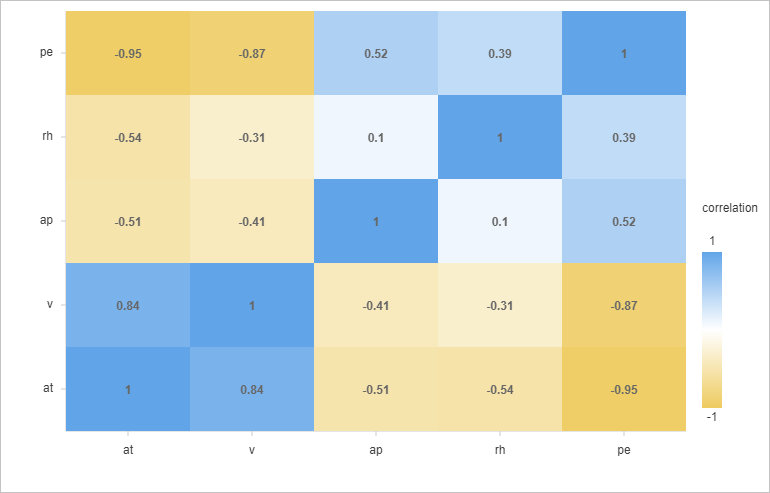This topic describes how use a preset template of Machine Learning Studio to build a model to predict the output power of a power plant.

## Background information

In the following sample experiment, the power generation data of a combined cycle power plant is used to build a model to predict the output power. This shows the application of machine learning in the industrial production. The output power of a wind energy converter determines the electrical energy that one generator can produce. If you can accurately predict the output power of the generator, you can evaluate and carry out the power production plan to reduce the waste of resources.

## Dataset

The dataset of the combined cycle power plant that is used in the following sample experiment is a dataset that University of California, Irvine (UCI) provides in Machine Learning Repository. For more information, see Combined Cycle Power Plant Data Set. The dataset contains 9,568 data entries. Each data entry includes the AT, V, AP, RH, and PE fields, which indicate the measurements of the temperature, pressure, humidity, pressure intensity, and output power in sequence. The following figure shows the data that is used in the experiment:## Procedure

1. Go to the Machine Learning Studio console.
1. Log on to the PAI console.
2. In the left-side navigation pane, choose Model Training > Studio-Modeling Visualization.
3. On the PAI Visualization Modeling page, find the project in which you want to create an experiment and click Machine Learning in the Operation column.2. Create an experiment.
1. In the left-side navigation pane, click Home.
2. In the Templates section, click Create below Electric Field Output Power Forecast.
3. In the New Experiment dialog box, set the experiment parameters. You can use default values for the parameters.
Parameter Description
Name The name of the experiment. Default value: Electric Field Output Power Forecast. The name must be 1 to 32 characters in length. Enter a name that meets this requirement, for example, Output power prediction.
Project The project in which you want to create the experiment. You cannot change the value of this parameter.
Description The description of the experiment. Default value: enter Predict the output of the generator power to better evaluate the arrangement of power production plan, to avoid waste of resources.
Save To The directory for storing the experiment. Default value: My Experiments.
4. Click OK.
5. Optional:Wait about 10 seconds. Then, click Experiments in the left-side navigation pane.
6. Optional:Click Output power prediction_XX under My Experiments. The canvas of the experiment appears.
My Experiments is the directory for storing the experiment that you created and Output power prediction_XX is the name of the experiment. In the experiment name, _XX is the ID that the system automatically creates for the experiment.
7. View the components of the experiment on the canvas, as shown in the following figure. The system automatically creates the experiment based on the preset template.Area No. Description
1 The Correlation Coefficient Matrix-1 component measures the impact of each feature on the output power. After the experiment is run, you can right-click the Correlation Coefficient Matrix-1 component on the canvas and select View Analytics Report to view the impact of each feature on the output power.
2 The Split-1 component divides the dataset into a training dataset and a prediction dataset at the ratio of 8 to 2.
3 The Linear Regression-1 component performs regression modeling.
4 The Prediction-1 component predicts output power based on the prediction dataset. The Regression Model Evaluation-1 component evaluates the prediction accuracy of the model provides.
3. Run the experiment and view the result.
1. In the top toolbar of the canvas, click Run.
2. After the experiment is run, right-click the Correlation Coefficient Matrix-1 component on the canvas and select View Analytics Report.
3. In the Correlation Coefficient Matrix dialog box, select Show Correlation to view the impact of each feature on the output power.The preceding figure shows that the temperature has the greatest impact on the output power, then the pressure, then the humidity, and then the pressure intensity.
4. Right-click the Linear Regression-1 component on the canvas and choose Model Option > Show Model to view the weight of each feature in the prediction result.
5. Right-click the Regression Model Evaluation-1 component on the canvas and select View Analytics Report to view the model evaluation indexes.